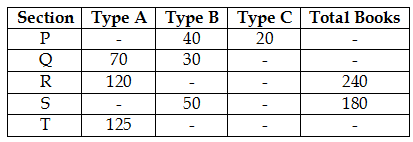## PREPARATORY QUESTIONS

### DATA INTERPRETATION (TABLE)

#### PREPARATORY SET-13 (TABLE)

Direction (Qs.1 to 5): Table given below shows the number of 3 types of books (A, B and C) in five different sections of a library.

Some values are deliberately missed, you are supposed to find those values and then solve the questions given below.Question No : 1

If number of type C books in sections Q, R, S and T is 50, 40, 30 and 30 respectively, then what will be the ratio of total books in sections Q, R and S together to the number of type B books in sections P, Q and R together?

(1) 13 : 9

(2) 19 : 5

(3) 13 : 5

(4) 19 : 9

(5) None of these

Question No : 2

If a total type B book in section R is 60, then find the approximate difference between the percent of type C books in section R and percent of type B books in section S.

(1) 10

(2) 5.56

(3) 2.78

(4) 12.7n8

(5) None of these

Question No : 3

Find that in how many sections the total number of type A books is more than 70, if average of type A books in sections Q, R and T is 25 books more than that of in sections P and S and ratio of type A books in section P and S is 3 : 5.

(1) 2

(2) 3

(3) 1

(4) 4

(5) None of these

Question No : 4

Find that what is the total number of books in section Q, if the number of type C books in section Q is (125/6)% of the total books in section R?

(1) 300

(2) 250

(3) 200

(4) 150

(5) None of these

Question No : 5

Find the number of type C books in section T, if total books in section R is 20% more than that of in section T and number of type B books in section T is 50% more than that of in section Q.

(1) 45

(2) 30

(3) 60

(4) 50

(5) None of these